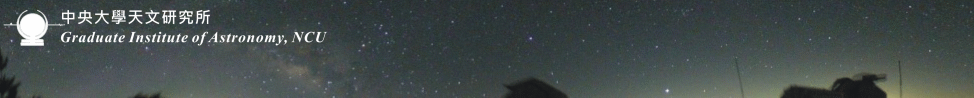# 演講資訊

## Muchen Chiu (Shanghai Normal University)"Alternative cosmology and f(R) gravity"

### 摘要：

The observation of the accelerated expansion of the Universe is one of the most mysterious puzzles in modern cosmology. In this talk, I will focus on one candidate theory which may contain the explanation, f(R) theory, which is the simplest model of modified gravity that can ensure a stable accelerated expansion. I will show that if we allow the Hubble parameter, H(a), in f(R) gravity to oscillate around ΛCDM, those f(R) models with weff closer to -1 do not necessarily provide a better alternative to ΛCDM. I will also show a new approach that allows us to solve for linearized perturbations in f(R) theories exactly, by reducing the equations for the perturbations into fourcoupled first order equations governing the Newtonian potential, Ψ, and the curvature potential, Φ. According to this new method, there is little difference between the exact solutions and that of the quasi- Newtonian approach even up to k = 10c-1H0, which disagree with previous studies. Finally, I will also discuss the conditions for a stable f(R) model, and show that the ultimate fate of viable f(R) models, including Hu and Sawicki's bi-power models, might be to end with the time coordinate becoming imaginary.

回上一頁